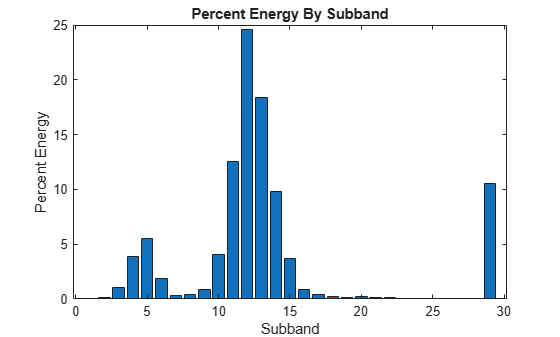# tqwtmra

Tunable Q-factor multiresolution analysis

## Syntax

``mra = tqwtmra(wt,n)``
``mra = tqwtmra(wt,n,QualityFactor=qf)``
``tqwtmra(___)``

## Description

````mra = tqwtmra(wt,n)` returns the tunable Q-factor wavelet multiresolution analysis (MRA) for the TQWT analysis, `wt`, obtained with the default quality factor of 1.```

example

````mra = tqwtmra(wt,n,QualityFactor=qf)` uses the quality factor `qf` in obtaining the tunable Q-factor MRA. `qf` must match the value used in obtaining `wt` from `tqwt`.```
````tqwtmra(___)` with no output arguments plots the tunable Q-factor wavelet MRA in a new figure. For complex-valued data, the real part is plotted in the first color in the MATLAB® color order matrix and the imaginary part is plotted in the second color. This syntax does not support multidimensional MRAs.```

## Examples

collapse all

Load an ECG signal. Obtain the TQWT of the signal down to level 6 with a quality factor of 2.

```load wecg wt = tqwt(wecg,QualityFactor=2,Level=6);```

Obtain the tunable Q-factor MRA of the signal.

`mra = tqwtmra(wt,length(wecg),QualityFactor=2);`

Plot the original signal and the lowpass subband.

```plot(wecg) hold on plot(mra(end,:),linewidth=2) hold off axis tight legend(["Original","Lowpass"])```Confirm the sum along the rows of the MRA equals the original signal.

```mraSum = sum(mra,1); max(abs(mraSum(:)-wecg(:)))```
```ans = 9.4369e-16 ```

Load the Kobe earthquake data. Obtain the tunable Q-factor wavelet transform of the data using a quality factor of 3.

```load kobe qf = 3; wt = tqwt(kobe,QualityFactor=qf);```

Identify the subbands that contain at least 15% of the total energy. Note that the last element of `wt` contains the lowpass subband coefficients.

```EnergyBySubband = cellfun(@(x)norm(x,2)^2,wt)./norm(kobe,2)^2*100; idx15 = EnergyBySubband >= 15; bar(EnergyBySubband) title("Percent Energy By Subband") xlabel("Subband") ylabel("Percent Energy")```Obtain a multiresolution analysis and sum those MRA components corresponding to previously identified subbands.

```mra = tqwtmra(wt,numel(kobe),QualityFactor=qf); ts = sum(mra(idx15,:)); plot([kobe ts']) axis tight legend("Original Data","Large Energy Components",... Location="NorthWest") xlabel("Time (s)")```## Input Arguments

collapse all

Tunable Q-factor wavelet transform, specified as a cell array. The elements of `wt` contain the wavelet subband and lowpass coefficients. `wt` is expected to be the output of `tqwt`.

Data Types: `single` | `double`
Complex Number Support: Yes

Original signal length in samples, specified as a positive integer. If the original signal length `n` is odd, `n` is extended to `n+1` to obtain the MRA and the final sample is removed before returning the MRA.

Data Types: `single` | `double`

Quality factor, specified as a real-valued scalar greater than or equal to 1. If unspecified, the quality factor defaults to 1.

Example: `wt = tqwtmra(qt,2024,QualityFactor=1.5)` specifies a quality factor of 1.5.

Data Types: `single` | `double`

## Output Arguments

collapse all

Multiresolution analysis, returned as an array. `mra` is an Ns-by-N-by-C-by-B array where Ns denotes number of subbands in the tunable Q-factor wavelet transform ordered by decreasing center frequency, N is the number of signal samples in time, C is the number of channels, and B is the batch size.

Data Types: `single` | `double`

 Selesnick, Ivan W. “Wavelet Transform With Tunable Q-Factor.” IEEE Transactions on Signal Processing 59, no. 8 (August 2011): 3560–75. https://doi.org/10.1109/TSP.2011.2143711.